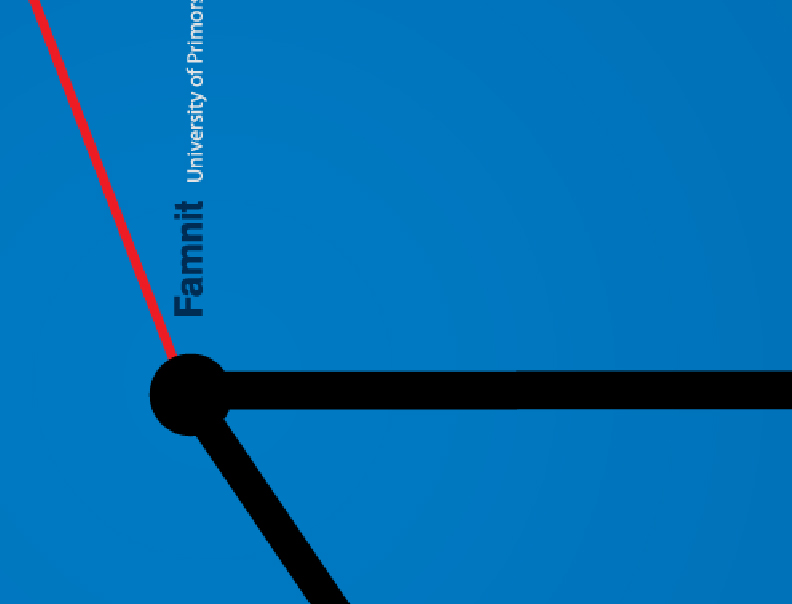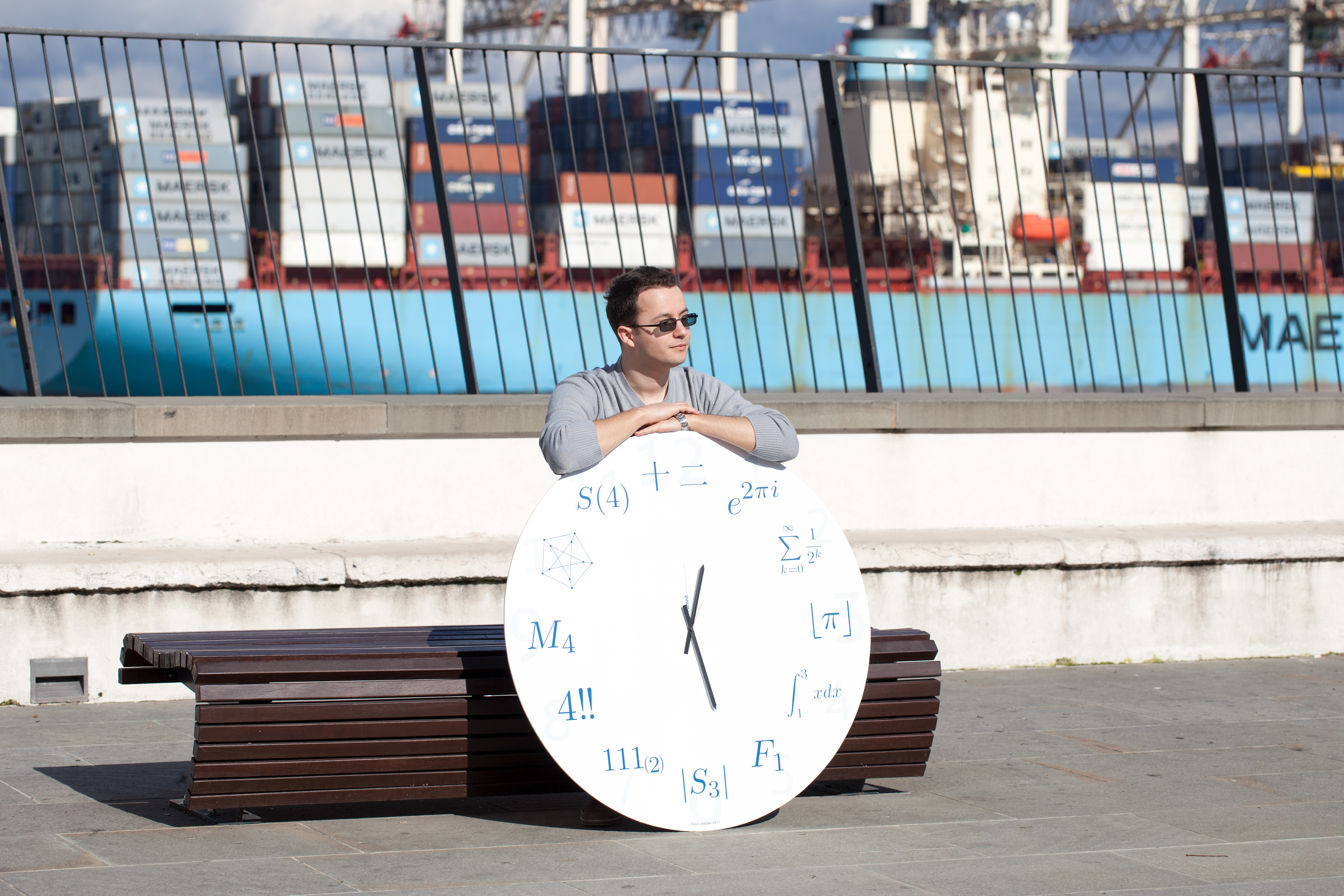Univerza na Primorskem Fakulteta za matematiko, naravoslovje in informacijske tehnologijeSI | EN# Raziskovalni matematični seminar - Arhiv

 2020 2019 2018 2017 2016 2015 2014 2013 2012 2011 2010
 1 2 3 4 5 6 7 8 9 10 11 12
30.05.2011 10:00 Seminarska soba v Galebu
Lecturer: Alexander Vasilyev

Title: Communicability Graph and Community Structures in Complex Networks
Abstract: Estrada and Hatano proposed an algorithm for the detection of community structure in complex networks using the concept of network communicability.The communities are defined as the cliques of a “communicability graph”, which has the same set of nodes as the complex network and links determined by the communicability function. Then, the problem of finding the network communities is transformed to an all-clique problem of the communicability graph. In addition, we extend here the concept of the communicability to account for the strength of the interactions between the nodes by using the concept of inverse temperature of the network. We develop an algorithm to manage the different degrees of overlapping between the communities in a complex network. Finally, we amend this algorithm by eliminating the subjectivity of choosing degree of overlapping and including an additional check of the fitness of detected communities. We show that this amendment can detect some communities which remain undetected by the original Estrada and Hatano’s algorithm.

23.05.2011 10:00 Seminarska soba v Galebu
Lecturer: Anna Klymenko

Title: The Coxeter Graph
Abstract: According to Lovasz conjecture, every finite connected vertex-transitive graph contains a Hamiltonian cycle except K2, Petersen, Coxeter graph and theirs truncations. We will prove that the Coxeter graph has no Hamiltonian cycle. We will also present some well-know properties of this remarkable graph.

16.05.2011 10:00 Seminarska soba v Galebu
Lecturer: dr. Boštjan Brešar ( FNM, Univerza v Mariboru and IMFM, Ljubljana )

Title: Median graphs and algebras
Abstract: In this talk we present a strong connection between certain ternary algebras, called median algebras, that enjoy three simple axioms, and the class of median graphs. The latter are defined as the graphs in which for any triple of vertices the three intervals between pairs of the triple share exactly one vertex. Median graphs are the most important class in metric graph theory, and appear in several applications in Computer science, mathematical biology and elsewhere.
We select a small portion of numerous characterizations and properties of median graphs, with an emphasis on some recent results.

16.05.2011 9:00 Seminarska soba v Galebu
Lecturer: dr. Matjaž Kovše ( FNM, Univerza v Mariboru and IMFM, Ljubljana )

Title: Steiner index of a graph
Abstract: Given a subset H of k vertices in a connected graph G the k-Steiner tree problem is that of finding a connected subgraph of G that contains all the k vertices and has the minimum number of edges among all such subgraphs. The number of edges in this subgraph is denoted by sG(H). It is well known that for k3, it is generally NP-hard to compute sG(H). The k-th Steiner index of a graph G is defined as the sum of all values sG(H) where H ranges over all subsets of k vertices of G. For k=2 this definition coincides with the definition of well known and well studied Wiener index. Hamming graphs are the Cartesian products of complete graphs, and their isometric subgraphs are called partial Hamming graphs. A polynomial time algorithm for computing the k-th Steiner index of partial Hamming graphs will be presented. The main ingredient will be the canonical metric representation of a graph.

09.05.2011 10:00 Seminarska soba v Galebu
Lecturer: dr. Ted Dobson ( Mississippi State University, Department of Mathematics and Statistics )

Title: The isomorphism classes of all generalized petersen graphs
Abstract:The generalized Petersen graphs GP(n, k) were introduced by Watkins in 1969, and since then have been studied extensively. For an ordered pair (n, k), where 1 k n 1, k = n/2, the generalized Petersen graph GP(n, k) has vertex set Z2×Zn and edge set {(1, i)(1, i+1), (0, ki)(0, ki+ k), (0, i)(1, i) : i Zn}. Many well-known graphs are generalized Petersen graphs, with GP(5, 2) being the Petersen graph itself, GP(4, 1) being the skeleton of the three dimensional cube, GP(10, 2) being the skeleton of the dodecahedron, and GP(10, 3) is the Desargues graph (the Levi or incidence graph of the Desargues configuration). In this talk, we determine necessary and sufficient conditions for two generalized Petersen graphs GP(n, k) and GP(n, ℓ) to be isomorphic. The conditions are that either k ≡ ±ℓ (mod n) or that kℓ ≡ ±1 (mod n).